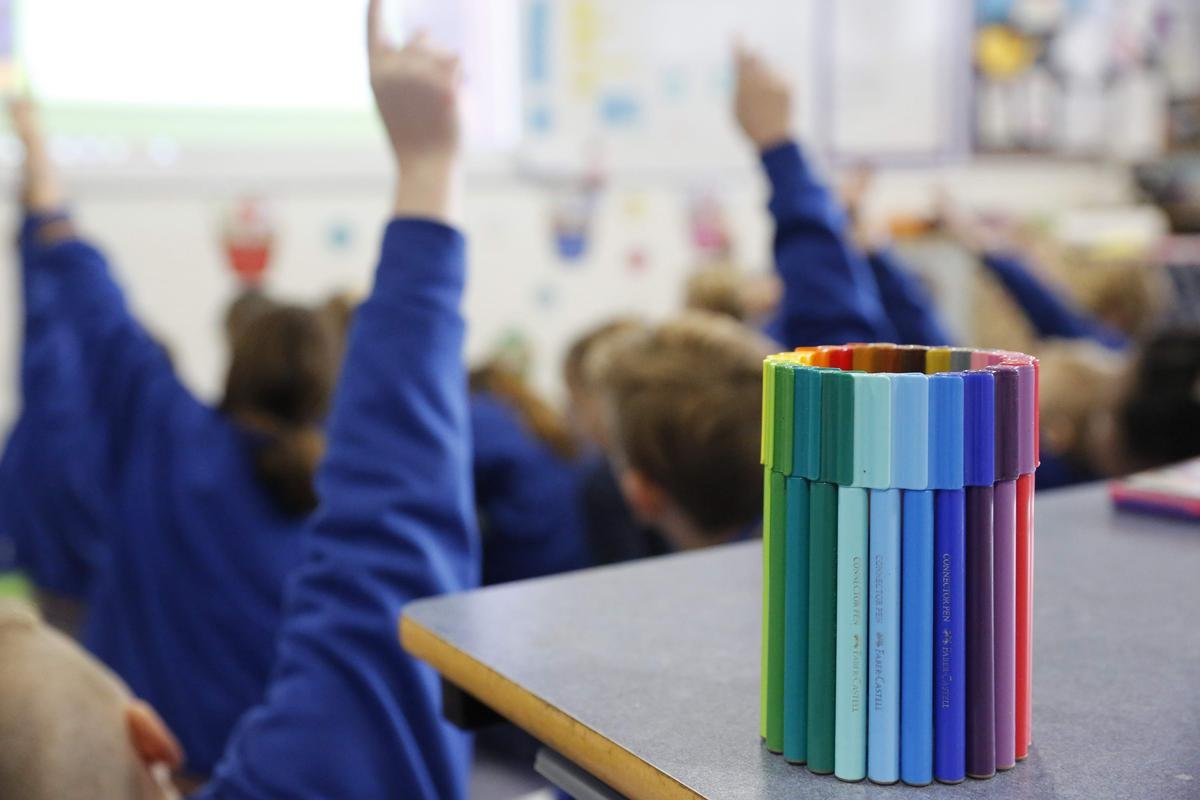# Mathematics Overview

Children will explore the following content:

They will be offered a range of multi-model learning experiences directed at their point of need based on ongoing assessment and the Victorian Curriculum.

## Number and Algebra

Students recognise the properties of prime, composite, square and triangular numbers and determine sets of these numbers.

They solve problems that involve all four operations with whole numbers and describe the use of integers in everyday contexts.

Students locate fractions and integers on a number line and connect fractions, decimals and percentages as different representations of the same number. They solve problems involving the addition and subtraction of related fractions. Students calculate a simple fraction of a quantity and calculate common percentage discounts on sale items, with and without the use of digital technology.

They make connections between the powers of 10 and the multiplication and division of decimals. Students add, subtract and multiply decimals and divide decimals where the result is rational.

Students write number sentences using brackets and order of operations, and specify rules used to generate sequences involving whole numbers, fractions and decimals.

They use ordered pairs of integers to represent coordinates of points and locate a point in any one of the four quadrants on the Cartesian plane.

## Measurement and Geometry

Students relate decimals to the metric system and choose appropriate units of measurement to perform a calculation. They solve problems involving length and area, and make connections between capacity and volume.

Students interpret a variety of everyday timetables.

They solve problems using the properties of angles and investigate simple combinations of transformations in the plane, with and without the use of digital technology.

Students construct simple prisms and pyramids.

## Statistics and Probability

Students interpret and compare a variety of data displays, including displays for two categorical variables. They analyse and evaluate data from secondary sources.

Students compare observed and expected frequencies of events, including those where outcomes of trials are generated with the use of digital technology.

They specify, list and communicate probabilities of events using simple ratios, fractions, decimals and percentages.